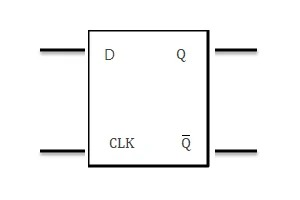﻿

# D Flip-Flop

## What is a D Flip-Flop?

### Definition

A D (or Delay) Flip Flop (Figure 1) is a digital electronic circuit used to delay the change of state of its output signal (Q) until the next rising edge of a clock timing input signal occurs.The truth table for the D Flip Flop is shown in Figure 2.### What is the D Flip Flop used for?

The D Flip Flop acts as an electronic memory component since the output remains constant unless deliberately changed by altering the state of the D input followed by a rising clock signal.

### Where are D Flip Flops used?

The D Flip Flop is a building block shift registers. For example, by cascading eight D Flip Flops in sequence, a byte (8-bits) of information can be stored after 8 clock cycles.

### What else can they be used for?

By connecting the inverting output of the D Flip Flop to the D input, a simple divide by two circuit is created i.e. the D output changes state at half the frequency of the clock signal. By cascading D flip flops and through appropriate design of external combinational logic gates, a countdown timer can be created.

Find a term alphabetically: